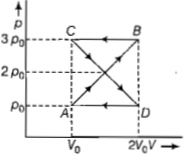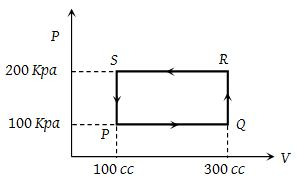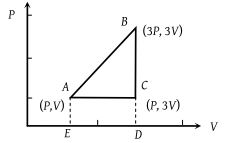You’ve reached the end of your free Videos limit.
#30 | Solved Example: 13
(Physics) > Thermodynamics
Related Practice Questions :

A thermodynamic system undergoes cyclic process ABCDA as shown in figure. The work done by the system in the cycle is(a) ρoV

(b) 2ρoVo

(c) ρoVo/2

(d)zero

Complete Question Bank + Test Series
Complete Question Bank

Difficulty Level:

If Q, E and W denote respectively the heat added, change in internal energy and the work done in a closed cyclic process, then

(a) W=0                                  (b) Q=W=0

(c) E=0                                   (d) Q=0

Complete Question Bank + Test Series
Complete Question Bank

Difficulty Level:

In a cyclic process, the internal energy of the gas -

(1) Increases

(2) Decreases

(3) Remains constant

(4) Becomes zero

Complete Question Bank + Test Series
Complete Question Bank

Difficulty Level:

A thermodynamic system is taken through the cycle PQRSP process. The net work done by the system is -(1) 20 J

(2) – 20 J

(3) 400 J

(4) – 374 J

Complete Question Bank + Test Series
Complete Question Bank

Difficulty Level:

An ideal gas is taken around ABCA as shown in the above P-V diagram. The work done during a cycle is(1) 2PV

(2) PV

(3) 1/2PV

(4) Zero